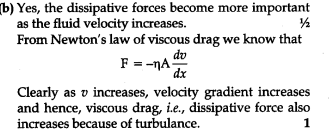# In deriving Bernoulli's equation, we equated the work done on the fluid in the tube to its change in the potential and kinetic energy

In deriving Bernoulli’s equation, we equated the work done on the fluid in the tube to its change in the potential and kinetic energy.
(a) How does the pressure change as the fluid moves along the tube if dissipative forces are present ?
(b) Do the dissipative forces become more important as the fluid velocity increases ? Discuss qualitatively.

While deriving Bernoulli’s equation, we say that Decrease in pressure energy per second = increase in K.E./sec + increase in P.E./sec Consider that viscous forces are absent. Thus as the fluid flows from lower to upper edge there is a fall of pressure energy due to the fall of pressure. If dissipating forces are pressent, then a part of this pressure energy will be used in overcoming these forces during the flow of fluid. Hence there shall be greater drop of pressure as the fluid moves along the tube.